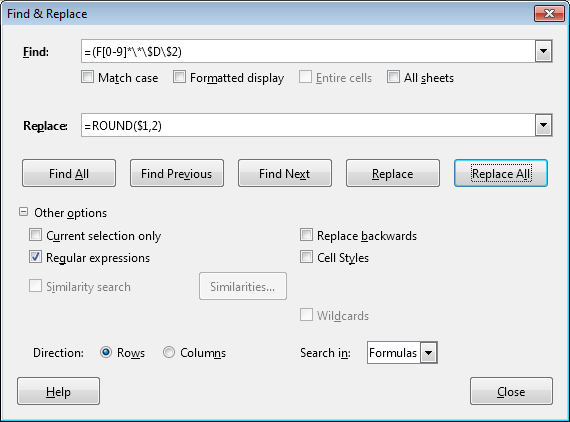# calc find & replace for formulas

I have a spreadsheet with a lot of rows. After being created, I have found that I need to change the formulas in all the cells. For example, I have a formula as follows: =F37*\$D\$2

What I need to do is change this to the following: =round(F37*\$D\$2,2)

Is there a way I can do this with find & replace???

Thanks,
Rich Ramik

It seems to me, Rich, that you’re looking for something like thisHere the first asterisk means “any digits” and the second (with a backslash) - the multiplication sign